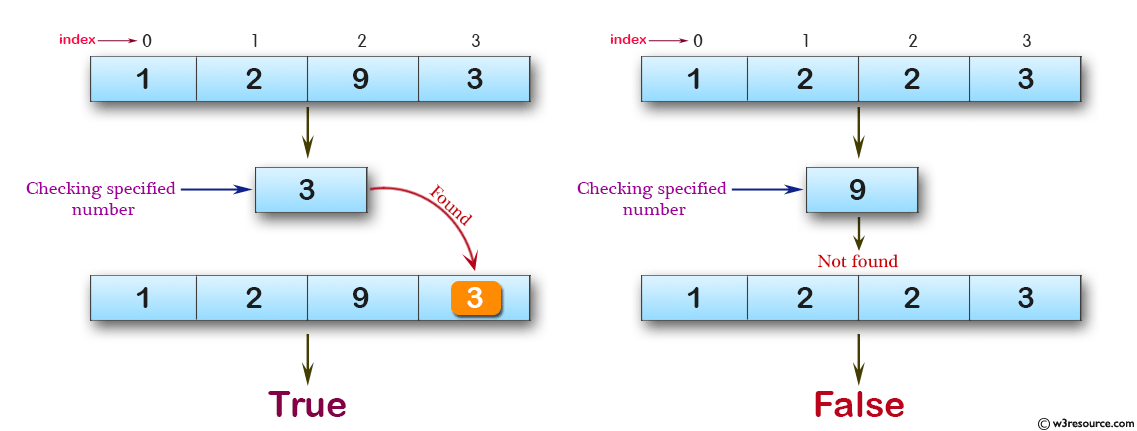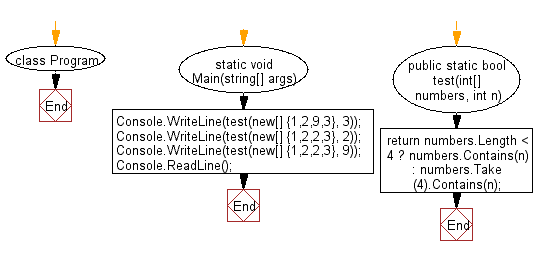# C# Sharp Basic Algorithm Exercises: Check a specified number is present in a given array of integers

## C# Sharp Basic Algorithm: Exercise-32 with Solution

Write a C# Sharp program to check a specified number is present in a given array of integers.

Pictorial Presentation:Sample Solution:-

C# Sharp Code:

``````using System;
using System.Linq;
namespace exercises
{
class Program
{
static void Main(string[] args)
{
Console.WriteLine(test(new[] {1,2,9,3}, 3));
Console.WriteLine(test(new[] {1,2,2,3}, 2));
Console.WriteLine(test(new[] {1,2,2,3}, 9));
}
public static bool test(int[] numbers, int n)
{
if (numbers.Contains(n))
return true;
return false;
}
}
}
```
```

Sample Output:

```True
True
False
```

Flowchart:C# Sharp Code Editor:

Improve this sample solution and post your code through Disqus

What is the difficulty level of this exercise?

Test your Programming skills with w3resource's quiz.

﻿